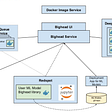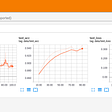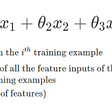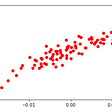# Logistic Regression

## What are the different types of logistic regression?

1. Binary logistic regression is the statistical technique used to predict the relationship between the dependent variable (Y) and the independent variable (X), where the dependent variable is binary in nature. For example, the output can be Success/Failure, 0/1 , True/False, or Yes/No. This is the type of logistic regression that we’ve been focusing on in this post.
2. Multinomial logistic regression is used when you have one categorical dependent variable with two or more unordered levels (i.e two or more discrete outcomes). It is very similar to logistic regression except that here you can have more than two possible outcomes. For example, let’s imagine that you want to predict what will be the most-used transportation type in the year 2030. The transport type will be the dependent variable, with possible outputs of train, bus, tram, and bike (for example).
3. Ordinal logistic regression is used when the dependent variable (Y) is ordered (i.e., ordinal). The dependent variable has a meaningful order and more than two categories or levels. Examples of such variables might be t-shirt size (XS/S/M/L/XL), answers on an opinion poll (Agree/Disagree/Neutral), or scores on a test (Poor/Average/Good).

• Logistic regression is easier to implement, interpret, and very efficient to train.
• It makes no assumptions about distributions of classes in feature space.
• It can easily extend to multiple classes(multinomial regression) and a natural probabilistic view of class predictions.
• Logistic regression works well for cases where the dataset is linearly separable.

• Logistic regression fails to predict a continuous outcome.
• If the number of observations is lesser than the number of features, Logistic Regression should not be used, otherwise it may lead to overfitting.
• Logistic Regression requires average or no multicollinearity between independent variables.
• Logistic regression may not be accurate if the sample size is too small.

## Penalty Term

1. L1 regularization: It adds an L1 penalty that is equal to the absolute value of the magnitude of coefficient, or simply restricting the size of coefficients. For example, Lasso regression implements this method.
2. L2 Regularization: It adds an L2 penalty which is equal to the square of the magnitude of coefficients. For example, Ridge regression and SVM implement this method.
3. Elastic Net: When L1 and L2 regularization combine together, it becomes the elastic net method, it adds a hyperparameter.

## Conclusion

--

--

--

Love podcasts or audiobooks? Learn on the go with our new app.

## YOLO where have all the airplanes gone! Check the satellite image will you## Google trained a trillion-parameter AI language model## Airbnb’s End-to-End ML Platform## Creation of a Feed-forward Neural Network -FashionMNIST## Review of Deep Learning Algorithms for Image Semantic Segmentation## Explain sentiment prediction with LIME## Neural network training on free cloud GPU. Google Colab, Pytorch, Drive and TensorboardX.## Contours in Images## Multivariate Linear Regression## Linear Regression Assumptions## Batch Gradient Descent and Simple Linear Regression## Linear Regression